# How to Calculate the Tenure for Staff in Months or Years in Excel

For HR assistant or department secretary, calculate the tenure for staff in their company or department is one normal task in their daily work, so acquire the skill of calculating tenure in excel is necessary. This article will provide a simple formula to calculate tenure, the final value can be displayed in months or years depends on your demands.

Prepare a simple table. Suppose some of the list already quit. Some are still working for this company.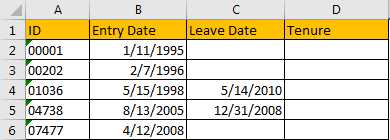## Calculate the Tenure for Staff in Months

To calculate the tenure for a staff, we can use DATEDIF function. It is used for returning the Years/Months/Dates between two dates. Please see the general formula for DATEDIF function.

=DATEDIF(start_date,end_date,unit)

Now let’s start to calculate the tenure in months.

Step 1: in C2, enter the formula =DATEDIF(B2,NOW(),”M”). In this formula, B2 is start date, as there is no end date, so we enter NOW() to calculate the months between entry date till now, M is month unit.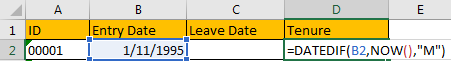Step 2: Click Enter to get result.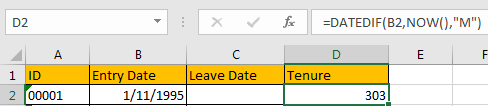Step 3: If there is end date parameter, for example, for ID 01036, leave date is 5/14/2010, we can enter the formula =DATEDIF(B4,C4,”M”) .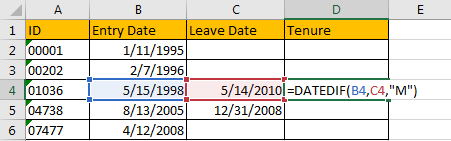Step 4: Click Enter to get result.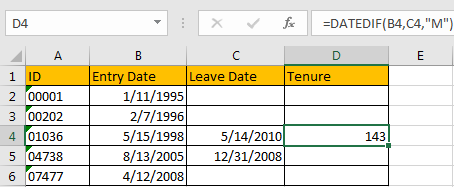## Calculate the Tenure for Staff in Years

We just need to change the unit from M to Y.

Step 1: In D2, enter the formula =DATEDIF(B2,NOW(),”Y”). Then click Enter.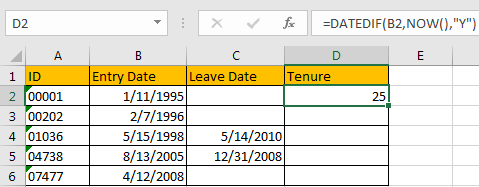Step 2: In D4, enter the formula =DATEDIF(B4,C4,”Y”). Then click Enter.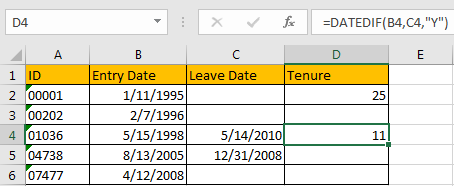## Calculate the Tenure for Staff in Years and Months

If we want to show the Tenure like XX years and XX months, we need to update the formula. Please be aware that when calculating Months, Years of the dates should be ignored.

Step 1: In D2, enter the formula =DATEDIF(B2,NOW(),”Y”)&” years, “&DATEDIF(B2,NOW(),”YM”)&” months “. Then click Enter. Verify that 25 years and 3 months are displayed.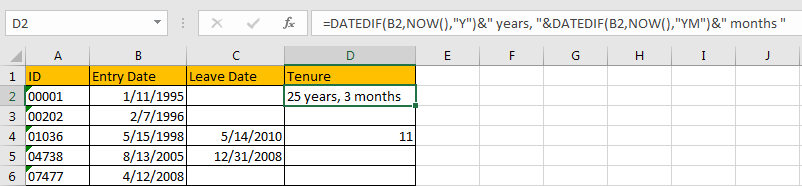Step 2: In D4, enter the formula =DATEDIF(B4,C4,”y”)&” years, “&DATEDIF(B4,C4,”ym”)&” months “.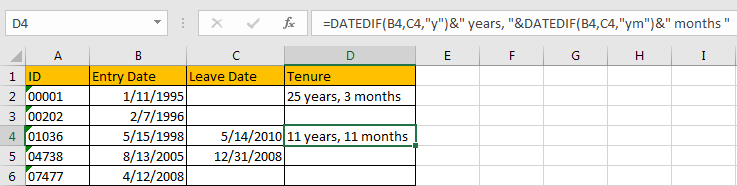Through above steps, we can find that the tenure is more accurate if we set smaller unit in formula. So, you can calculate tenure by Years and Months, Months and Dates or Years and Dates per your demands accordingly.

### Related Functions

• Excel DATEDIF function
The Excel DATEDIF function returns the number of days, months, or years between tow dates.The syntax of the DATEDIF function is as below:=DATEDIF (start_date,end_date,unit)…

Related Posts

Calculate Number of Hours between Two Times

Calculating the difference between two times might be a valuable statistic for subsequent computations or averages, whether you're producing a time sheet for staff or recording personal exercises. While Excel has a plethora of complex functions, including date and time ...

Calculate Loan Interest in Given Year

When you borrow money, you are supposed to repay it gradually. Lenders, on the other hand, want to be compensated for their services and the risk they incur by lending you money. That is, you will not just repay the ...

Calculate Interest Rate for Loan

The interest rate is the fee charged by a lender to a borrower and is expressed as a percentage of the principal—the lent amount. The interest rate on a loan is often expressed as an annual percentage rate, abbreviated as ...

Calculate Interest for Given Period

Using the IPMT function in Excel, we can compute the interest payment on any loan. This step-by-step tutorial will guide Excel users of all skill levels through the process to calculate interest for given period. Finally, the formula: =IPMT(B3/12,1,B5,-B2) The ...

How To Use Excel GCD Function

This post will guide you how to use Excel GCD function with syntax and examples in Microsoft excel. Description The Excel GCD function Returns the greatest common divisor of two or more integers. So you can use the GCD function ...

Calculate A Ratio From Two Numbers In Excel

In elementary mathematics, a ratio is a connection or comparison between two or more integers. For example, ratios are often expressed as ":" to demonstrate the relationship between two numbers. You would think that manually calculating a ratio from two ...

How To Use Excel RRI Function

This post will guide you how to use Excel RRI function with syntax and examples in Microsoft excel. Description The Excel RRI function Returns an equivalent interest rate for the growth of an investment. So you can use the RRI ...

CAGR Formula Examples in Excel

CAGR in Excel is a formula that calculates the compound annual growth rate for any invested amount over the specified years or timeframe. Although there is no direct function in Excel that can help us identify the CAGR value, there ...

Build Hyperlink With VLOOKUP in Excel

You might have come across a task in which you were assigned to build hyperlinks, which seems very easy, and if you are new to excel or don't have enough experience with it, then you might wonder about doing this ...

Break ties with helper COUNTIF and column

Suppose you got a task to adjust the values that contain the ties; what would be your first attempt to break the ties of the given value? If you are wondering about doing this task manually, let me add that ...

Sidebar